Click to Chat

1800-1023-196

+91-120-4616500

CART 0

• 0

MY CART (5)

Use Coupon: CART20 and get 20% off on all online Study Material

ITEM
DETAILS
MRP
DISCOUNT
FINAL PRICE
Total Price: Rs.

There are no items in this cart.
Continue Shopping```Basic Conceptsf(x) = a0 + a1x1 + a2x2 + … anxn, where a1, a2, ……… an are constants, is called a polynomial.

If a1 ai Î R where i = 0, 1, 2, ……, n, then we call it a polynomial of a real variable with real coefficients.

If an ¹ 0 then we say degree of the polynomial is n. In case of complex coefficients it is called a complex polynomial.

We shall discuss more about the properties of the polynomials. At present, let us look at a particular case i.e. n = 2. When n = 2, then we call it a quadratic polynomial.

f(x) = a0 + a1 + a2x2 = 0 is called quadratic equation.

The general form of the quadratic equation is represented by

ax2 + bx + c = 0.

where a, b, c Î R and a ¹ 0. If all the three coefficients are non-zero then

ax2 + bx + c = 0 is called the complete form of the quadratic equation.

Now, ax2 + bx + c = 0 can be written as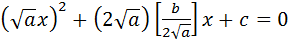[If a < 0 we can multiply both sides of the equation by (–1) to make ‘a’ positive].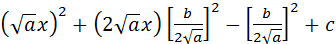= 0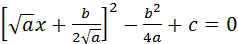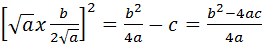taking square root on both sides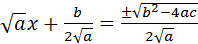Þ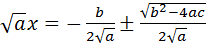=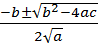Þ x =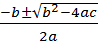so finally we have two solution (Let us denote them by a and b).

a =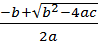and b =These two solutions of a quadratic equation are called the roots of the equation.

Sum and product of the roots

Let ax2 + bx + c = 0 be a quadratic equation whose roots are a and b then

a + b =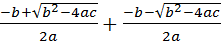=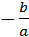Hence, Sum of roots =Next, product of roots i.e. a.b

=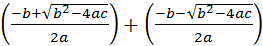=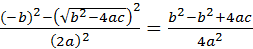product of roots =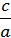.

Note:

Let a and b are the given roots. Then (x – a)(x – b) = 0 must be a equation of 2nd degree in x with a and b as solutions(roots)

x2 – (a + b) x + ab = 0

x2 – (sum of the roots)x + product of the roots = 0

If you multiply the above equation by any constant (¹ 0), the resultant equation will also be quadratic in nature and have the same roots as parent equation. You may know that, in general for a polynomial equation of degree. i.e.

a0 xn + a1xn–1 + …… + an = 0, a0 ¹ 0                       …… (i)

If the roots are a1, a2 …… + an, the polynomial equation can be written as

a0 (x – a) ……… (x – an) = 0

a0 [xn – S1xn–1 + S2xn–2 …… + (–1)r Sr xn–r +………] = 0        …… (ii)

Where Sr denotes sum of the products of roots taken r at a time eg.

S3 = a1a2a3 + a2a3a1 + a3a1a2 …… Comparing the coefficients in (i) and (ii) of different powers of x, we observe that

S1 = a1/a0, S2 = a2/a0 and in general Sr = (–1)r ar/a0

Illustration:

Let us take an example of a cubic polynomial equation for verifying the      above deductions.

a0x3 + a1x2 + a2x + a3 = 0, a0 ¹ 0                                          … (i)

Solution:

If a1, a2 and a3 are its three roots then above equations can be written        as

a0 (x – a1)(x – a2)(x – a3) = 0

Þ     a0 [x3–a1+a2+a3)x2 + (a1a2+a2a3+a3a1)x – a1a2a3] = 0        … (ii)

from (i) and (ii) comparing coefficients of different powers we get

a1 + a2 + a3 =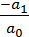= S1

a1a2 + a2a3 + a3a1 =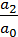= S2

and   a1a2a3 =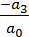= S3

To read more, Buy study materials of Quadratic Equation comprising study notes, revision notes, video lectures, previous year solved questions etc. Also browse for more study materials on Mathematics here.
```### Course Features

• 731 Video Lectures
• Revision Notes
• Previous Year Papers
• Mind Map
• Study Planner
• NCERT Solutions
• Discussion Forum
• Test paper with Video Solution